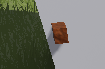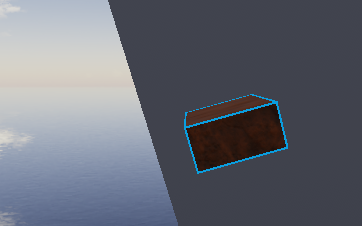# How do I make a Dragger?

I’m making a module script for a game that requires some sort of building system. Roblox Studio’s method of placing parts is perfect for what I’m doing. How can I make something similar?

1. What happened to DraggerService? I searched through the docs and api and found DraggerService, but cannot access it in game. Being able to use DraggerService (for increments and other things) with Draggers will solve my issue.

2. How do I do the same thing Roblox is doing? I’ve been through the forum and found some posts about it, but none of them (except this one, but I can’t load the .rbxm file as apparently its corrupted.) presents a solid solution I can use.Here’s a snippet of my current code/module, made with some help from existing forum posts:

``````local function ConvertWithIncrement(v3: Vector3, increment: number, size, unitNormal)
local x, y, z = v3.X, v3.Y, v3.Z
if increment > 0 then
x = math.sign(x)*((math.abs(x) - math.abs(x) % increment) + (size.X % increment))
y = math.sign(y)*((math.abs(y) - math.abs(y) % increment) + (size.Y % increment))
z = math.sign(z)*((math.abs(z) - math.abs(z) % increment) + (size.Z % increment))
end
return Vector3.new(x, y, z)
end

function Dragger:MouseMove(unitRay: Ray, params: RaycastParams)

local rayStart = unitRay.Origin
local rayDirection = unitRay.Direction

if self.Target then
if not params then
params = RaycastParams.new()
params.FilterType = Enum.RaycastFilterType.Exclude
params.IgnoreWater = true
params.FilterDescendantsInstances = {self.Target}
end

local hit = game.Workspace:Raycast(rayStart, rayDirection * self.RaycastRange, params)
if hit and hit.Instance then
local pos = hit.Position

-- offset by the face of the target
local size
if self.Target.ClassName == "Model" then
local normClone = self.Target:Clone()
normClone.Parent = game.ReplicatedStorage
normClone:PivotTo(CFrame.new(0,0,0))
_, size = normClone:GetBoundingBox()
else
size = self.Target.Size
end
pos += hit.Normal * (size/2)

-- grid locking
pos = ConvertWithIncrement(pos, self.Increment, size, hit.Normal)

local c = CFrame.new(pos, pos + hit.Normal)

if self.Target.ClassName == "Model" then
self.Target:PivotTo(c)
else
self.Target.CFrame = c
end
end
else
warn("No target was found.")
end
end
``````

The script works the best when the snap increment is 0. Otherwise, the position is snapped “globally”, and so the part can clip into the part it is being placed on.
Another issue is that unlike in Roblox’s dragger system, the part’s orientation doesn’t match up with the part its being placed on.

Current:Snap and orientation are off
Expected:Snap and orientation matches that of the canvas part

EDIT:
I looked through again and found this. How do you use/find the “new framework”?

1 Like

Have you checked this out?

Yes, its a really neat system of using the Dragger object, except there still isn’t a way to change the increment and other things Roblox’s draggers can use because it still uses a Dragger object.

If i can fix the file corruption issue and open the file i think i can just look at roblox’s code to see how their dragger works. I dont know why it says its corrupted or if im doing something wrong

Or is it possible for you to send me your studio’s MoveDragger.rbxm file (if that is the file roblox uses for dragging objects in the scene editor)?

Then again, I don’t really know if you can just “import” the .rbxm file from the builtinplugins folder, but when I open the file with notepad it shows a bunch of…not code

EDIT:
This post also shows that there is a lua file somewhere that developers can use to see how the draggers work. Is this the BuiltInPlugin/MoveDragger.rbxm file?

YESS okay I found a good solution

I went and revisited this, and realised i can just use the other part as the ‘canvas’

Heres the completed code if anyone cares:

``````local Dragger = {ClassName = "Dragger"}
Dragger.__index = Dragger

function Dragger.new()
local self = {}
self.Target = nil

-- to be set manually
self.Increment = 0
self.RaycastRange = 100

setmetatable(self, Dragger)
return self
end

function Dragger:MouseDown(target: "Part/Model to move")
self.Target = target
end

function Dragger:CalcCanvas(canvas)
local canvasSize = canvas.Size

local up = Vector3.new(0, 1, 0)
local back = -self.SurfaceVector

local dot = back:Dot(Vector3.new(0, 1, 0))
local axis = (math.abs(dot) == 1) and Vector3.new(-dot, 0, 0) or up

local right = CFrame.fromAxisAngle(axis, math.pi/2) * back
local top = back:Cross(right).unit

local cf = canvas.CFrame * CFrame.fromMatrix(-back*canvasSize/2, right, top, back)
local size = Vector2.new((canvasSize * right).magnitude, (canvasSize * top).magnitude)

return cf, size
end
function Dragger:CalcPlacementCFrame(position, rotation, surfaceVector, canvas)
self.SurfaceVector = surfaceVector
local cf, size = self:CalcCanvas(canvas)

local tSize = self.Target.Size
if self.Target.ClassName == "Model" then tSize = self.Target.PrimaryPart.Size end

local modelSize = CFrame.fromEulerAnglesYXZ(0, rotation, 0) * tSize
modelSize = Vector3.new(math.abs(modelSize.x), math.abs(modelSize.y), math.abs(modelSize.z))

local lpos = cf:pointToObjectSpace(position);
local size2 = (size - Vector2.new(modelSize.x, modelSize.z))/2

if size2.x < 0 or size2.y < 0 then return end

local x = math.clamp(lpos.x, -size2.x, size2.x);
local y = math.clamp(lpos.y, -size2.y, size2.y);

local g = self.Increment
if (g > 0) then
x = math.sign(x)*((math.abs(x) - math.abs(x) % g) + (size2.x % g))
y = math.sign(y)*((math.abs(y) - math.abs(y) % g) + (size2.y % g))
end

return cf * CFrame.new(x, y, -modelSize.y/2) * CFrame.Angles(-math.pi/2, rotation, 0)
end

function Dragger:MouseMove(unitRay: Ray, params: RaycastParams)

local rayStart = unitRay.Origin
local rayDirection = unitRay.Direction

if self.Target then
if not params then
params = RaycastParams.new()
params.FilterType = Enum.RaycastFilterType.Exclude
params.IgnoreWater = true
params.FilterDescendantsInstances = {self.Target}
end

local hit = game.Workspace:Raycast(rayStart, rayDirection * self.RaycastRange, params)
if hit and hit.Instance and hit.Instance ~= game.Workspace.Terrain then
local pos = hit.Position

local surface
local dotY = hit.Normal:Dot(Vector3.new(0,1,0))
local dotX = hit.Normal:Dot(Vector3.new(1,0,0))
local dotZ = hit.Normal:Dot(Vector3.new(0,0,1))
if (math.abs(dotY) >= 0.9) then
if (dotY < 0) then
surface = Enum.NormalId.Bottom
else
surface = Enum.NormalId.Top
end
elseif (math.abs(dotX) >= 0.9) then
if (dotX < 0) then
surface = Enum.NormalId.Left
else
surface = Enum.NormalId.Right
end
else
if (dotZ < 0) then
surface = Enum.NormalId.Front
else
surface = Enum.NormalId.Back
end
end

local c = self:CalcPlacementCFrame(pos, 0, Vector3.FromNormalId(surface), hit.Instance)

if not c then return end

if self.Target.ClassName == "Model" then
self.Target:PivotTo(c)
else
self.Target.CFrame = c
end
end
else
warn("No target was found.")
end
end

function Dragger:MouseUp()
self.Target = nil
end

return Dragger

``````
1 Like

This topic was automatically closed 14 days after the last reply. New replies are no longer allowed.